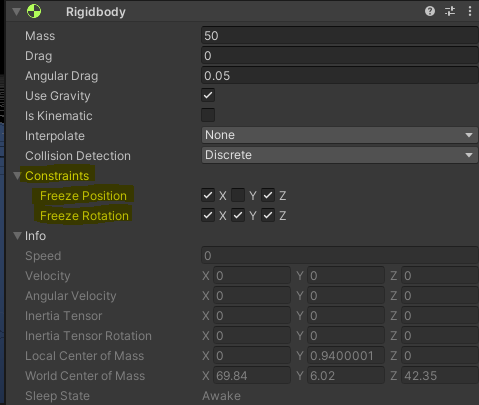# Unity DOTS Physics constraint linear and angular x,y,z

Hi,

I am trying to constraint my entity physic as per MonoBehaviour RigidbodyI have found some posts that helped me, but I cant make this work. Here is what I have done so far…

This is a component that contains bool3 for the constraint:

``````[GenerateAuthoringComponent]
public struct PhysicsConstraintComponent : IComponentData
{
public bool3 linearConstrains;
public bool3 angularConstrains;
}
``````

This is the system that creates the PhysicJoint:

``````        Entities
.ForEach((Entity entity, in PhysicsConstraintComponent constraint, in Translation translation, in Rotation rotation) => {

var rigidTransform = new RigidTransform( rotation.Value, translation.Value);

var body = new BodyFrame(rigidTransform);

PhysicsJoint joint = new PhysicsJoint
{
BodyAFromJoint = body,
};

FixedList128<Constraint> constraints = new FixedList128<Constraint> {
new Constraint
{
ConstrainedAxes = constraint.linearConstrains,
Type = ConstraintType.Linear,
Min = 0,
Max = 0,
SpringFrequency = Constraint.DefaultSpringFrequency,
SpringDamping = Constraint.DefaultSpringDamping
},
new Constraint
{
ConstrainedAxes = constraint.angularConstrains,
Type = ConstraintType.Angular,
Min = 0,
Max = 0,
SpringFrequency = Constraint.DefaultSpringFrequency,
SpringDamping = Constraint.DefaultSpringDamping
}
};

joint.SetConstraints(constraints);

``````        PhysicsConstrainedBodyPair pcbp = new PhysicsConstrainedBodyPair(entity, default, false);